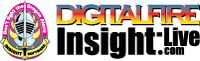•The secret to cool bodies and glazes is a lot of testing.
•The secret to know what to test is material and chemistry knowledge.
•The secret to learning from testing is documentation.
•The place to test, do the chemistry and document is an account at https://insight-live.com
•The place to get the knowledge is https://digitalfire.com

Sign-up at https://insight-live.com today.

# Texas GK Ball Clay

 Oxide Analysis Formula CaO 0.40% 0.023 MgO 0.30% 0.024 K2O 0.10% 0.003 Na2O 0.10% 0.005 TiO2 1.20% 0.049 Al2O3 31.37% 1.000 SiO2 53.55% 2.897 Fe2O3 0.80% 0.016 LOI 12.19 Oxide Weight 285.55 Formula Weight 325.19

## XML for Import into INSIGHT

<?xml version="1.0" encoding="UTF-8"?> <material name="Texas GK Ball Clay" descrip="" searchkey="" loi="12.19" casnumber="70694-09-6"> <oxides> <oxide symbol="CaO" name="Calcium Oxide, Calcia" status="" percent="0.400" tolerance=""/> <oxide symbol="MgO" name="Magnesium Oxide, Magnesia" status="" percent="0.300" tolerance=""/> <oxide symbol="K2O" name="Potassium Oxide" status="" percent="0.100" tolerance=""/> <oxide symbol="Na2O" name="Sodium Oxide, Soda" status="" percent="0.100" tolerance=""/> <oxide symbol="TiO2" name="Titanium Dioxide, Titania" status="" percent="1.200" tolerance=""/> <oxide symbol="Al2O3" name="Aluminum Oxide, Alumina" status="U" percent="31.370" tolerance=""/> <oxide symbol="SiO2" name="Silicon Dioxide, Silica" status="" percent="53.550" tolerance=""/> <oxide symbol="Fe2O3" name="Iron Oxide, Ferric Oxide" status="" percent="0.800" tolerance=""/> </oxides> <volatiles> <volatile symbol="LOI" name="Loss on Ignition" percent="12.190" tolerance=""/> </volatiles> </material>